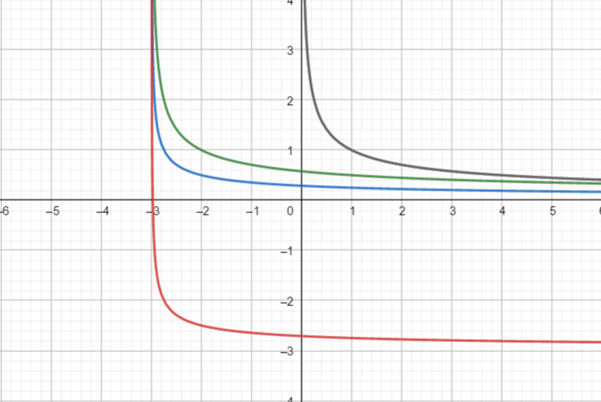# To graph the functionson the same screen and how they related to the graph in part (a). The functions are given below: ( a ) y 1 = 1 x ( b ) y 2 = 1 x + 3 ( c ) y 3 = 1 2 x + 3 ( d ) y 4 = 1 2 x + 3 − 3### Precalculus: Mathematics for Calcu...

6th Edition
Stewart + 5 others
Publisher: Cengage Learning
ISBN: 9780840068071### Precalculus: Mathematics for Calcu...

6th Edition
Stewart + 5 others
Publisher: Cengage Learning
ISBN: 9780840068071

#### Solutions

Chapter 2.5, Problem 72E
To determine

## To graph the functionson the same screen and how they related to the graph in part (a).The functions are given below:  (a) y1=1x(b)y2=1x+3(c)y3=12x+3(d)y4=12x+3−3

Expert Solution

### Explanation of Solution

Given information:

The function are:

(a) y1=1x(b)y2=1x+3(c)y3=12x+3(d)y4=12x+33

Graph :

the graph obtained as:Interpretation: from the above graph it is observed that for part(b) shiftthe graph of part (a) to the left 3 units.

For part (c) shift the graph of part (a) to the left 3 units and shrink vertically by a factor of 12 .

For part (d) shift the graph of part (a) to the left 3 units and shrink vertically by a factor of 12 and then shift 3 unit downwards.

### Have a homework question?

Subscribe to bartleby learn! Ask subject matter experts 30 homework questions each month. Plus, you’ll have access to millions of step-by-step textbook answers!